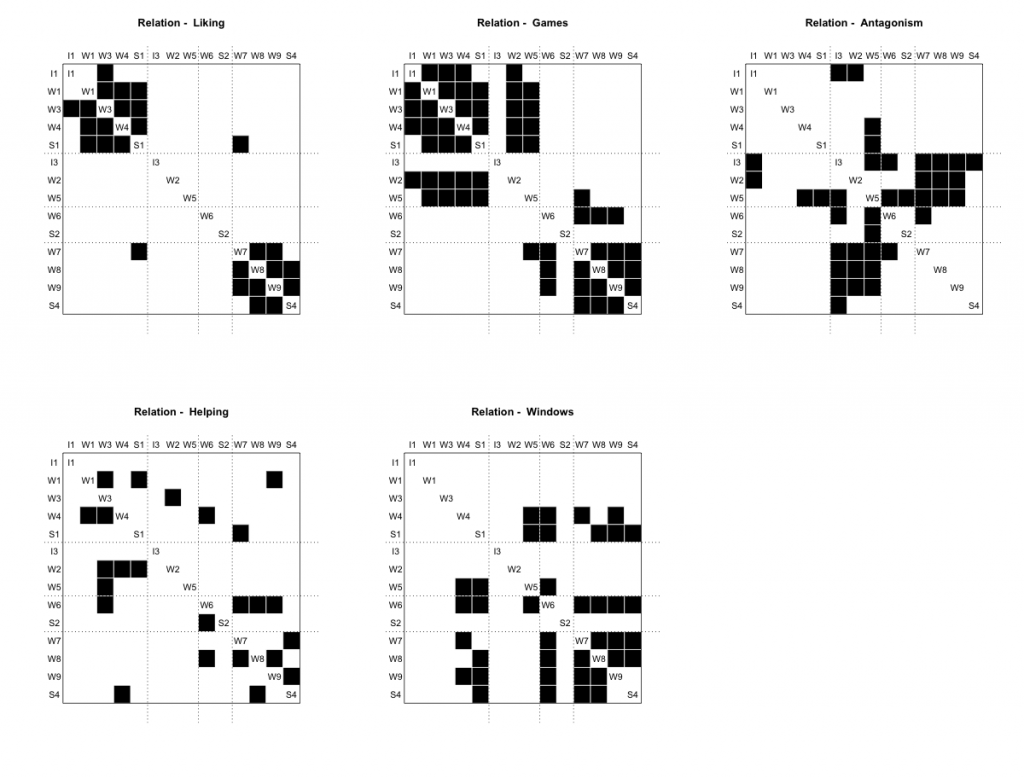Want to share your content on R-bloggers? click here if you have a blog, or here if you don't.

In network analysis, blockmodels provide a simplified representation of a more complex relational structure. The basic idea is to assign each actor to a position and then depict the relationship between positions. In settings where relational dynamics are sufficiently routinized, the relationship between positions neatly summarizes the relationship between sets of actors. How do we go about assigning actors to positions? Early work on this problem focused in particular on the concept of structural equivalence. Formally speaking, a pair of actors is said to be structurally equivalent if they are tied to the same set of alters. Note that by this definition, a pair of actors can be structurally equivalent without being tied to one another. This idea is central to debates over the role of cohesion versus equivalence.

In practice, actors are almost never exactly structural equivalent to one another. To get around this problem, we first measure the degree of structural equivalence between each pair of actors and then use these measures to look for groups of actors who are roughly comparable to one another. Structural equivalence can be measured in a number of different ways, with correlation and Euclidean distance emerging as popular options. Similarly, there are a number of methods for identifying groups of structurally equivalent actors. The equiv.clust routine included in the sna package in R, for example, relies on hierarchical cluster analysis (HCA). While the designation of positions is less cut and dry, one can use multidimensional scaling (MDS) in a similar manner. MDS and HCA can also be used in combination, with the former serving as a form of pre-processing. Either way, once clusters of structurally equivalent actors have been identified, we can construct a reduced graph depicting the relationship between the resulting groups.

Yet the most prominent examples of blockmodeling built not on HCA or MDS, but on an algorithm known as CONCOR. The algorithm takes it name from the simple trick on which it is based, namely the CONvergence of iterated CORrelations. We are all familiar with the idea of using correlation to measure the similarity between columns of a data matrix. As it turns out, you can also use correlation to measure the degree of similarity between the columns of the resulting correlation matrix. In other words, you can use correlation to measure the similarity of similarities. If you repeat this procedure over and over, you eventually end up with a matrix whose entries take on one of two values: 1 or -1. The final matrix can then be permuted to produce blocks of 1s and -1s, with each block representing a group of structurally equivalent actors. Dividing the original data accordingly, each of these groups can be further partitioned to produce a more fine-grained solution.

Insofar as CONCOR uses correlation as a both a measure of structural equivalence as well as a means of identifying groups of structurally equivalent actors, it is easy to forget that blockmodeling with CONCOR entails the same basic steps as blockmodeling with HCA. The logic behind the two procedures is identical. Indeed, Breiger, Boorman, and Arabie (1975) explicitly describe CONCOR as a hierarchical clustering algorithm. Note, however, that when it comes to measuring structural equivalence, CONCOR relies exclusively on the use of correlation, whereas HCA can be made to work with most common measures of (dis)similarity.

Since CONCOR wasn’t available as part of the sna or igraph libraries, I decided to put together my own CONCOR routine. It could probably still use a little work in terms of things like error checking, but there is enough there to replicate the wiring room example included in the piece by Breiger et al. Check it out! The program and sample data are available on my GitHub page. If you have devtools installed, you can download everything directly using R. At the moment, the concor_hca command is only set up to handle one-mode data, though this can be easily fixed. In an earlier version of the code, I included a second function for calculating tie densities, but I think it makes more sense to use concor_hca to generate a membership vector which can then be passed to the blockmodel command included as part of the sna library.

#REPLICATE BREIGER ET AL. (1975)
#INSTALL CONCOR
devtools::install_github("aslez/concoR")

#LIBRARIES
library(concoR)
library(sna)

data(bank_wiring)
bank_wiring

#CHECK INITIAL CORRELATIONS (TABLE III)
m0 <- cor(do.call(rbind, bank_wiring))
round(m0, 2)

#IDENTIFY BLOCKS USING A 4-BLOCK MODEL (TABLE IV)
blks <- concor_hca(bank_wiring, p = 2)
blks

#CHECK FIT USING SNA (TABLE V)
#code below fails unless glabels are specified
blk_mod <- blockmodel(bank_wiring, blks\$block,
glabels = names(bank_wiring),
plabels = rownames(bank_wiring[]))
blk_mod
plot(blk_mod)


The results are shown below. If you click on the image, you should be able to see all the labels.